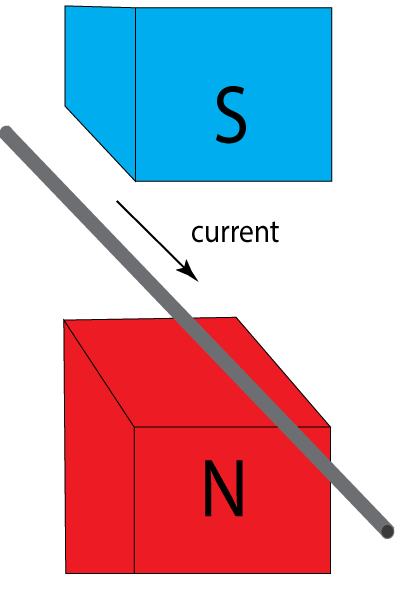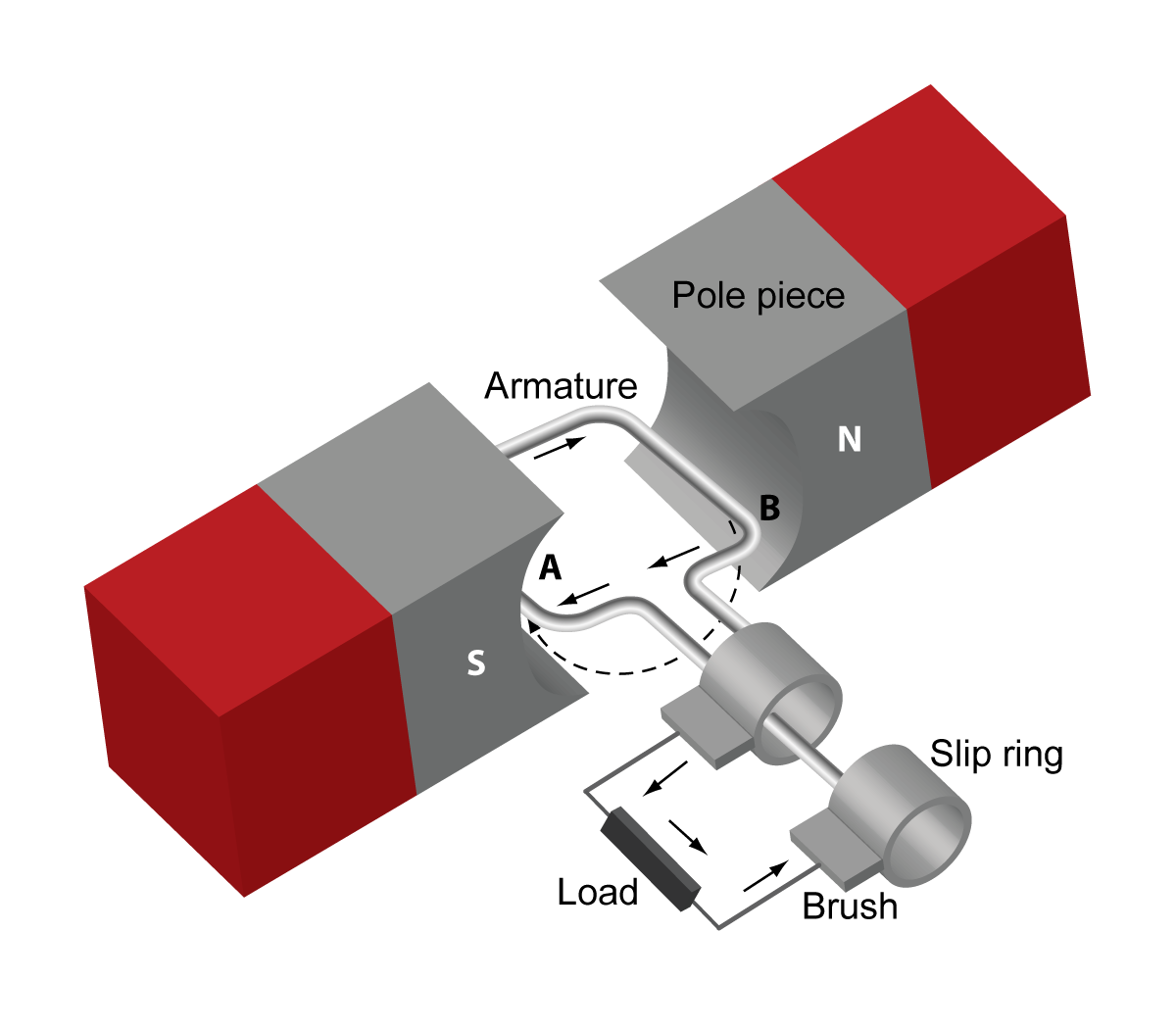1. Which of these diagrams shows the magnetic field lines around the north pole of a magnet?2. Which one of these could not have a magnetic field around it?

• A). Horseshoe magnet
• B). Electric cable
• C). Copper bar
• D). Compass

3-7. What are the missing words in this passage about the 'motor effect'?

 In the diagram shown, a wire has a current flowing though it and is placed between two magnets. There is ? an electric field a magnetic field a repulsion a field zone between the north and south poles pointing ? upwards downwards to the left to the right . This pushes the wire ? upwards downwards to the left to the right . If the direction of the current was reversed, the wire would be pushed ? upwards downwards to the left to the right . This force is only produced when the direction of the current is ? perpendicular parallel close similar to the direction of the field lines.8. Which one of these appliances does not use the effect described in the last question?

• A). Loudspeaker
• B). Motor
• C). Light bulb
• D). Relay

9. The force on a wire can be made stronger in several ways. Which one of these will not work?

• A). Increase the current
• B). Use stronger magnets
• C). Use a coil with many turns
• D). Make the wires thinner

10. If you push a metal bar quickly between 2 magnets it produces a voltage in the bar. What is this effect called?

• A). Induction
• B). Reduction
• C). Generation
• D). Production
11. The diagram shows an a.c. generator, made with a coil turning inbetween two magnets. A voltage is produced, which is used to heat a resistor (labelled the load in the diagram).What would happen if the coil was rotated faster?

• A). The resistor would stay the same temperature.
• B). The resistor would take more current but stay the same temperature.
• C). The voltage and temperture would increase
• D). The resistor would cool down.

12. Which of these methods will not generate a voltage?

• A). Rotate a coil in a magnetic field
• B). Rotate a magnet inside a coil
• C). Move a magnet past a wire
• D). Move a wire past a coil

13. A.C. generators are found in ...

• A). C.D. players
• B). Phone chargers
• C). Power stations
• D). Amplifiers